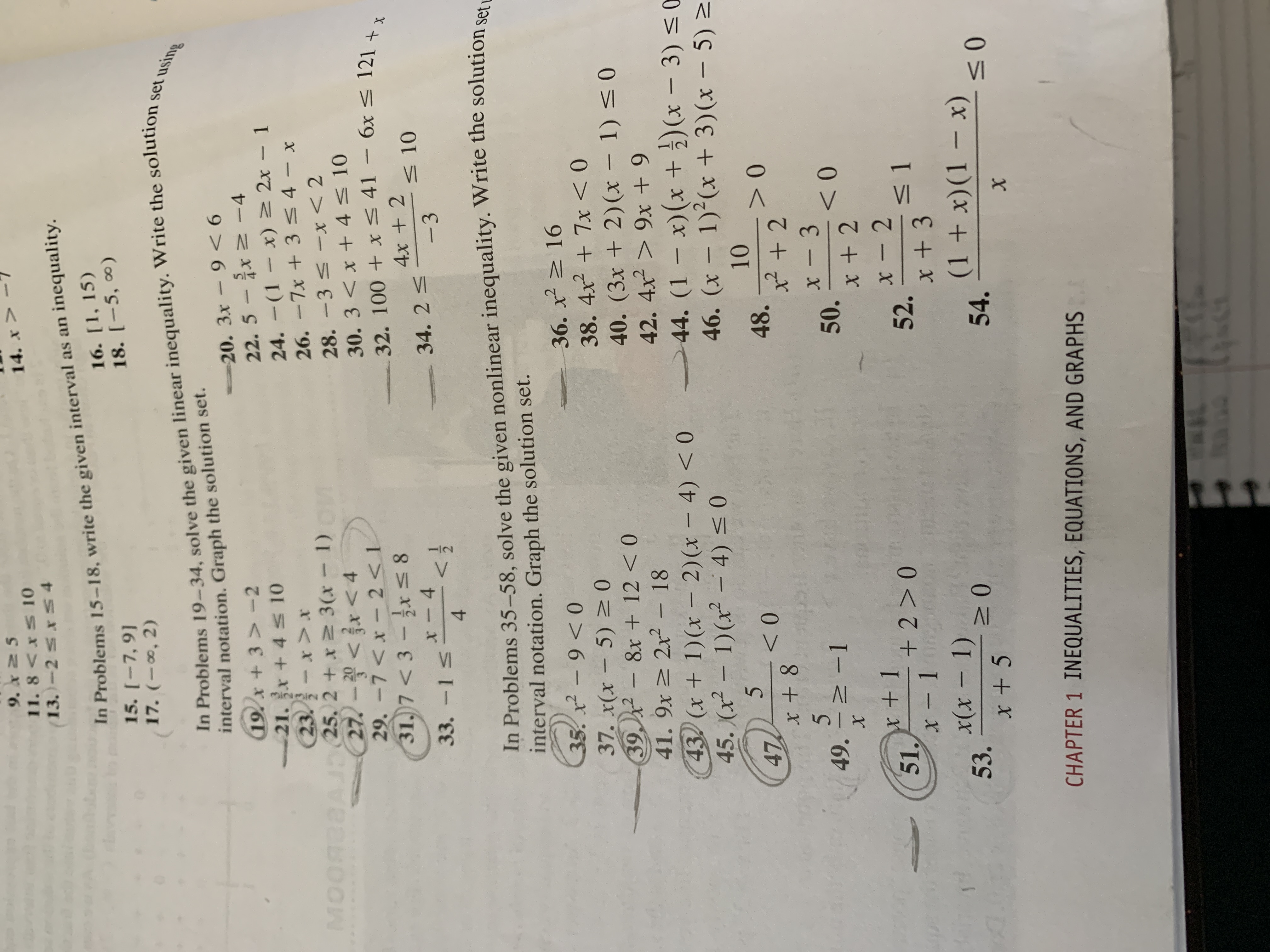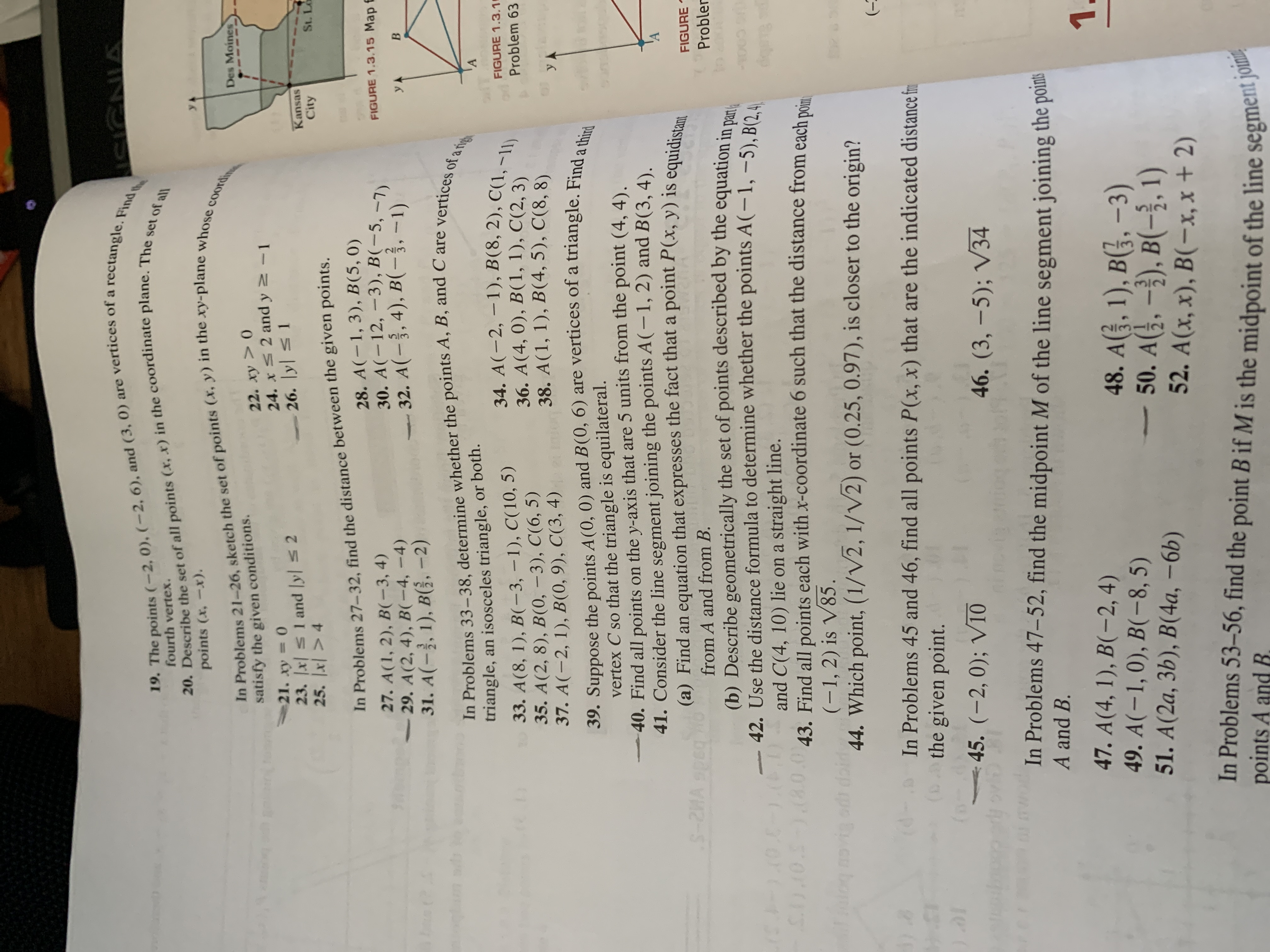# 9. x2 5 14. x > 11.8 -2 20. 3x- 9 < 6 22. 5 - x 2 -4 24. -(1 – x) > 2x – 1 26. -7x + 3<4 - x 21.x + 4 10 23.- x> x 25. 2 +x 2 3(x – 1) MOORBBA 28. -3 < -x< 2 27-< x< 4 30. 3 < x + 4 < 10 32. 100 + x< 41 – 6x < 121 + x 3. 29. -7 2x² – 18 43 (x + 1)(x – 2)(x – 4) < 0 45. (x² – 1)(x² – 4) < 0 40. (3x + 2)(x – 1) < 0 42. 4x > 9x + 9 44. (1 – x)(x +)(x – 3) <0 46. (x – 1)²(x + 3)(x – 5) = 47 5 x + 8 10 48. х — 3 50. 49. N-1 51. + 2 > 0 - 52. x + 3 x(x - 1) 53. (1 + x)(1 – x) 54. - x +5 х CHAPTER 1 INEQUALITIES, EQUATIONS, AND GRAPHS 19. The points (-2, 0). (-2, 6), and (3, 0) are vertices of a rectangle. Find the 20. Describe the set of all points (x, r) in the coordinate plane. The set of all In Problems 21-26, sketch the set of points (x, y) in the xy-plane whose coordin ISIGNIA fourth vertex. points (x, -x). Des Moines 22. ху > 0 24. x 2 and y 2-1 26. y 1 satisfy the given conditions. %3D 23. x s1 and yl s2 25. x| > 4 Kansas City St. Lo In Problems 27–32, find the distance between the given points. 28. A(-1, 3), B(5, 0) 30. A(-12, -3), B(-5, –7) 32. A(-3, 4), B(-3, -1) 27. A(1, 2), B(-3, 4) 29. A(2, 4), B(-4,-4) 31. A(-}, 1), B(}, -2) FIGURE 1.3.15 Map f In Problems 33-38, determine whether the points A, B, and C are vertices of atigh 'A triangle, an isosceles triangle, or both. 34. A(-2, -1), B(8, 2), C(1, -11) 36. A(4, 0), B(1, 1), C(2, 3) 38. A(1, 1), B(4, 5), C(8, 8). 33. A(8, 1), B(-3, –1), C(10, 5) 35. A(2, 8), B(0, –3), C(6, 5) 37. A(-2, 1), B(0, 9), C(3, 4) 39. Suppose the points A(0, 0) and B(0, 6) are vertices of a triangle. Find a third FIGURE 1.3.1 Problem 63 УА vertex C so that the triangle is equilateral. 40. Find all points on the y-axis that are 5 units from the point (4, 4). 41. Consider the line segment joining the points A(-1, 2) and B(3, 4). (a) Find an equation that expresses the fact that a point P(x, y) is equidistan S-2MA speg FIGURE from A and from B. Probler (b) Describe geometrically the set of points described by the equation in nam. 42. Use the distance formula to determine whether the points A(-1, -5), B(2,4, and C(4, 10) lie on a straight line. 010.-10.0.043. Find all points each with x-coordinate 6 such that the distance from each poim (-1,2) is V85. 44. Which point, (1/V2, 1/V2) or (0.25, 0.97), is closer to the origin? (- In Problems 45 and 46, find all points P(x, x) that are the indicated distance fm the given point. (o 45. (-2, 0); V10 46. (3, –5); V34 In Problems 47–52, find the midpoint M of the line segment joining the poins A and B. 1. 47. A(4, 1), B(-2, 4) 49. A(-1,0), B(-8, 5) 51. A(2a, 3b), B(4a, –6b) 48. A(}, 1), B(3. –3) 50. A(}, –}), B(-3, 1) 52. A(x, x), B(-x, x + 2) In Problems 53-56, find the point B if M is the midpoint of the line segment joinm points A and R.

Question

Looking for help for all of the questions with lines next to them.help_outlineImage Transcriptionclose9. x2 5 14. x > 11.8 -2 20. 3x- 9 < 6 22. 5 - x 2 -4 24. -(1 – x) > 2x – 1 26. -7x + 3<4 - x 21.x + 4 10 23.- x> x 25. 2 +x 2 3(x – 1) MOORBBA 28. -3 < -x< 2 27-< x< 4 30. 3 < x + 4 < 10 32. 100 + x< 41 – 6x < 121 + x 3. 29. -7 2x² – 18 43 (x + 1)(x – 2)(x – 4) < 0 45. (x² – 1)(x² – 4) < 0 40. (3x + 2)(x – 1) < 0 42. 4x > 9x + 9 44. (1 – x)(x +)(x – 3) <0 46. (x – 1)²(x + 3)(x – 5) = 47 5 x + 8 10 48. х — 3 50. 49. N-1 51. + 2 > 0 - 52. x + 3 x(x - 1) 53. (1 + x)(1 – x) 54. - x +5 х CHAPTER 1 INEQUALITIES, EQUATIONS, AND GRAPHS fullscreenhelp_outlineImage Transcriptionclose19. The points (-2, 0). (-2, 6), and (3, 0) are vertices of a rectangle. Find the 20. Describe the set of all points (x, r) in the coordinate plane. The set of all In Problems 21-26, sketch the set of points (x, y) in the xy-plane whose coordin ISIGNIA fourth vertex. points (x, -x). Des Moines 22. ху > 0 24. x 2 and y 2-1 26. y 1 satisfy the given conditions. %3D 23. x s1 and yl s2 25. x| > 4 Kansas City St. Lo In Problems 27–32, find the distance between the given points. 28. A(-1, 3), B(5, 0) 30. A(-12, -3), B(-5, –7) 32. A(-3, 4), B(-3, -1) 27. A(1, 2), B(-3, 4) 29. A(2, 4), B(-4,-4) 31. A(-}, 1), B(}, -2) FIGURE 1.3.15 Map f In Problems 33-38, determine whether the points A, B, and C are vertices of atigh 'A triangle, an isosceles triangle, or both. 34. A(-2, -1), B(8, 2), C(1, -11) 36. A(4, 0), B(1, 1), C(2, 3) 38. A(1, 1), B(4, 5), C(8, 8). 33. A(8, 1), B(-3, –1), C(10, 5) 35. A(2, 8), B(0, –3), C(6, 5) 37. A(-2, 1), B(0, 9), C(3, 4) 39. Suppose the points A(0, 0) and B(0, 6) are vertices of a triangle. Find a third FIGURE 1.3.1 Problem 63 УА vertex C so that the triangle is equilateral. 40. Find all points on the y-axis that are 5 units from the point (4, 4). 41. Consider the line segment joining the points A(-1, 2) and B(3, 4). (a) Find an equation that expresses the fact that a point P(x, y) is equidistan S-2MA speg FIGURE from A and from B. Probler (b) Describe geometrically the set of points described by the equation in nam. 42. Use the distance formula to determine whether the points A(-1, -5), B(2,4, and C(4, 10) lie on a straight line. 010.-10.0.043. Find all points each with x-coordinate 6 such that the distance from each poim (-1,2) is V85. 44. Which point, (1/V2, 1/V2) or (0.25, 0.97), is closer to the origin? (- In Problems 45 and 46, find all points P(x, x) that are the indicated distance fm the given point. (o 45. (-2, 0); V10 46. (3, –5); V34 In Problems 47–52, find the midpoint M of the line segment joining the poins A and B. 1. 47. A(4, 1), B(-2, 4) 49. A(-1,0), B(-8, 5) 51. A(2a, 3b), B(4a, –6b) 48. A(}, 1), B(3. –3) 50. A(}, –}), B(-3, 1) 52. A(x, x), B(-x, x + 2) In Problems 53-56, find the point B if M is the midpoint of the line segment joinm points A and R. fullscreen

## Expert Answer

### Want to see this answer and more?

Experts are waiting 24/7 to provide step-by-step solutions in as fast as 30 minutes!*

*Response times vary by subject and question complexity. Median response time is 34 minutes and may be longer for new subjects.
Tagged in© 2021 bartleby. All Rights Reserved.